BEM For Helmholtz Sound-Soft Scattering Problem Using NGSolve¶

We fix the notation that we will use from now on in this report, let $\Omega_{-}\subset \mathbb{R}^2$ be a bounded Liptschitz domain and $\Omega_{+}$ the complement of its closure in $\mathbb{R}^2$. We require $\Omega_{-}$ to be simply connected. Let us recall the Helmholtz PDE, that is gonna be the focus of our report: $$\Delta u + k^2 u=0$$

We will say that a function $u\in H^{1}_{loc}(\mathbb{R}^2\backslash B_R)$ is a radiating Helmholtz solution if for a given $k\in \mathbb{R}$: $$\Delta u + k^2 u=0 \;\forall x \in \mathbb{R}^2\backslash B_R$$ and $|\partial_r u - iku|=o(r^{-\frac{1}{2}})$ for $r\to \infty$.

Definition - Exterior Dirichlet Problem¶

Given $k\in\mathbb{N}$ and a function $g \in H^{\frac{1}{2}}(\partial\Omega_{-})$ we say that a function $u\in H^1_{loc}(\overline{\Omega_{+}})$ is solution to the Exterior Dirichlet Problem associated with $k$ and $g$ if, $$\Delta u + k^2 u = 0 \; in \; \Omega_{+}\\ \gamma^{+}u = g \;on\; \partial\Omega_{-}\\ u \; is \; radiating$$ where $\gamma^{+}$ is the trace operator from $H^1(\Omega_{+})\to H^{\frac{1}{2}}(\partial\Omega_{+})$, that is defined on $C^1(\overline{\Omega_{+}})$ and then extended by density.

Last we introduce the definition of Sound-Soft scattering problem that is gonna be the problem we aim to solve numerically and analytically in this report.

Definition¶

Given a function $u_{In}$ that is a radiating Helmholtz solution on $\partial\Omega_{-}$ we say that $u_{ST}\in H^{1}_{loc}(\overline{\Omega_{+}})$ is a solution to the sound soft scattering problem (SSSP) if: $$\Delta u_{ST} + k^2 u_{ST} = 0\; in \; \Omega_{+}\\ \gamma^{+}(u_{ST}+u_{IN})=0 \; on \; \Gamma\\ u_{ST}\; is \; radiating$$

The focus of this report will be to study the SSSP problems both analytically and numerically, in particular using a collocation BEM method using as computational toolbox the Netgen/NGSolve python interface more info. First of all we have to import all the library related to the Netgen/NGSolve environment, we also imported some elements from the native math library, numpy and matplotlib. Last but not least we import NGSolve special_functions library that has to be compiled separately and extend all the SLATEC functions as CoefficientFunctions more info.

In :
from ngsolve import * #Importing NGSolve library
import netgen.gui #Importing Netgen User Interface
import netgen.meshing as ms #Importing Netgen meshing library
import ngsolve.special_functions as sp #Importing NGSolve wrapper for SLATEC
from netgen.geom2d import SplineGeometry #Importing 2D geometry tools
#Importing std python library
from math import pi
import numpy as np
import matplotlib.pyplot as plt

Example - SSSP and Circular Scatterer¶

We want to consider a sound soft scattering problem where $\Omega$ is a circle of radius $\frac{1}{4}$ centered in the origin and $u_{In}$ is a planar wave defined as $$u_{in}(\vec{x}) = e^{ik(\vec{x}\cdot \vec{d})}, \;\; \vec{d}=\Big(\frac{1}{2},\frac{\sqrt{3}}{2}\Big)$$ In the following code we first define the geometry using Netgen 2D Geometry package and the we draw the planner wave $u_{In}$.

In :
geo = SplineGeometry()

########################
#  CIRCULAR SCATTERER  #
#######################
# In the following lines we define the circular scatterer

N = 40; #Number of segments in which we split the circle
pnts=[];
#We add points to the mesh, when N grows we get closer to a circle
for i in range(0,N):
pnts.append((0.25*cos(2*(i/N)*pi),0.25*sin(2*(i/N)*pi))) #Segments end points
#Mid points to define the correct spline
pnts.append((0.25*cos(2*(i/N)*pi+(pi/N)),0.25*sin(2*(i/N)*pi+(pi/N))))

#We connect the points to form a cirlce using 3 spline.
P = [geo.AppendPoint(*pnt) for pnt in pnts]
Curves = [[["spline3",P[i-1],P[i],P[i+1]],"c"+str(int((i-1)/2))] for i in range(1,2*N-1,2)]
Curves.append([["spline3",P[2*N-2],P[2*N-1],P],"c"+str(N-1)])
[geo.Append(c,bc=bc) for c,bc in Curves]

mesh = Mesh(geo.GenerateMesh(maxh=0.1)) #Meshing ...
#We define the polar coordinates
rho = sqrt(x**2+y**2);
theta = atan2(y,x)
Draw(theta,mesh,'theta')

#We here define a planar wave in the direction d=(0.5sqrt(3),0.5) with k=30.
# u(x) = exp(i(x°d)) = exp(ik*rho*|d|*cos(theta-pi/6))
k = 30;
lam = 1;

def PW(Angle):
pw = exp(1j*k*sqrt(x**2+y**2)*sqrt((0.5*sqrt(3))**2+0.25)*cos(theta-Angle));
return pw;
Uin = IfPos(rho-0.25,PW(pi/6),0)
Draw(Uin,mesh,'Uin',min=-1,max=1.0,autoscale=False)

Now we study a preliminary case, we assume $u_{In}$ to be a circular harmonic on $\partial \Omega_{+}$, i.e. $\gamma_{+}u_{In}(\theta) = e^{il\theta}$ for a fixed $l\in \mathbb{Z}$. In this case a solution of the SSSP is given by a convex combination of $\frac{H_l^{(j)}(kr)}{H_l^{(j)}(kR)}e^{il\theta}$, $j=1,2$. This because $\frac{H_l^{(j)}(kr)}{H_l^{(j)}(kR)}e^{il\theta}$ verifies the Helmholtz equation and therefore as well any linear combination of the above mentioned term verify the Helmholtz equation. Furthermore on $\partial\Omega{+}$ we have that $u_{In}+u_{St}$ is equal to: $$e^{il\theta} - \lambda\frac{H_l^{(1)}(kR)}{H_l^{(1)}(kR)}e^{il\theta} -(1-\lambda) \frac{H_l^{(2)}(kR)}{H_l^{(2)}(kR)}e^{il\theta} = e^{il\theta}-e^{il\theta}=0$$ Therefore we know that for a circular harmonic the solution has the form: $$u_{St}(\rho,\theta) = -\lambda\frac{H_l^{(1)}(k\rho)}{H_l^{(1)}(kR)}e^{il\theta} -(1-\lambda) \frac{H_l^{(2)}(k\rho)}{H_l^{(2)}(kR)}e^{il\theta}$$ we still have to meet the requirement that $u_{St}$ is a radiating Helmholtz solution, we expand the Henkel functions for a large argument as: $$H_l^{(j)}(z) = \sqrt{\frac{2}{\pi z}} e^{i(-1)^{j+1}(z-\frac{l\pi}{2}-\frac{\pi}{4})}$$ that only $-\lambda\frac{H_l^{(1)}(k\rho)}{H_l^{(1)}(kR)}e^{il\theta}$ is the radiating components and therefore we take $\lambda=1$. Now since we know that the Helmholtz equation is linear, such as the Dirichlet boundary condition and the radiating condition we can state that if we can decompose the $u_{In}$ in the sum of circular harmonics, i.e. $u_{In}(\rho,\theta) = \sum_{l\in \mathbb{Z}} a_l(\rho) e^{il\theta}$, we have a solution of the SSSP given by: $$\label{eq:HankelSolution}u_{St}(\rho,\theta) = \sum_{l\in \mathbb{Z}} -a_l(\rho)\frac{H_l^{(1)}(k\rho)}{H_l^{(1)}(kR)}e^{il\theta}$$

Let us consider a planar wave that propagates in the direction $(1,0)$ and that has wave number $k=3$, we can write its equation as: $$u(\vec{x}) = e^{ik(\vec{d}\cdot\vec{x})}$$ we can rewrite the above equation in polar coordinates as follow: $$u(\rho,\theta) = e^{ik\rho\cos(\theta)}$$ now we can put to good use the Jacobi-Anger expansion, i.e. $e^{i\rho\cos(\theta)} = J_0(\rho)+2\sum^{\infty}_{l=1} J_l(\rho)\cos(l\theta)$, to obtain: $$u(\rho,\theta) = J_0(k\rho)+2\sum^{\infty}_{l=1} J_l(k\rho)\cos(l\theta)$$ the usual form of the Jacobi-Anger is expansion is $e^{iz\cos(\theta)} = \sum^{\infty}_{l =-\infty} J_l(z)e^{il\theta}$, but using the identity $J_{-l}(z) = (-1)^l J_l(z)$ we can write it the form above. We use this representation because negative index Hankel function presents some problem when wrapped from SLATEC in NGSolve special functions. We now use the NGSolve toolbox to see if the series representation of $u_{In}$ is some how accurate, we then use the series decomposition of $u_{st}$ compute an approximation of $u_{St}$ and $u_{tot} = u_{In}+u_{St}$.

In :
#We define the MieSeries substituting the coeff of the Jacobi-Anger expansion in the
#series reppresentation of Ust

def MieSeriesPW(Angle):
#First term of the expansion
u = sp.jv(z=k*sqrt(x**2+y**2)*sqrt((0.5*sqrt(3))**2+0.25), v=0);
for l in range(1,50):
#Bessel portion of the expansion
J = sp.jv(z=k*sqrt(x**2+y**2)*sqrt((0.5*sqrt(3))**2+0.25), v=l)
a=cos(l*(theta-Angle)); #Jacobi-Anger coeff
t = (1j**l)*J*a; #Other term of the expansion
u = u+2*t; #Adding the term to the series
return u;
UMie = IfPos(rho-0.25,MieSeriesPW(pi/6),0)
Draw(UMie,mesh,'UMie',min=-1,max=1,autoscale=False) #Drawing the Mie series.

print("L2 error of Mie-Series rappresentation: ",abs(Integrate((UMie-Uin)**2,mesh)))
L2 error of Mie-Series rappresentation:  1.2379938758644846e-07
In :
def MieSerieST(Angle):
#Hankel portion of the expansion
h = sp.hankel1(z=k*sqrt(x**2+y**2),v=0)/sp.hankel1(z=k*0.25,v=0);
a = sp.jv(z=k*0.25, v=0) #Jacobi-Anger coeff
u = -1*a*h;
for l in range(1,50):
#Hankel portion of the expansion
h = sp.hankel1(z=k*sqrt(x**2+y**2),v=l)/sp.hankel1(z=k*0.25,v=l);
t = -2*(1j**l)*(sp.jv(z=k*0.25, v=l))*cos(l*(theta-Angle))*h; #Jacobi-Anger coeff
u = u+t; #Adding a new terms to the series
return u;
Ust = IfPos(rho-0.25,MieSerieST(pi/6),0)
Draw(Ust,mesh,'Ust',min=-1,max=1,autoscale=False)
Utot = Uin+Ust;
Draw(Utot,mesh,'Utot',min=-1,max=1,autoscale=False)
In :
from IPython.display import Video

Video("SSSP1.mp4")
Out:

Triangular Scatterer and Collocation BEM¶

In this section we focus on the SSSP where $\Omega_{-}$ is a generic polygon, in particular choose as $\Omega_{-}$ a triangle which has barycenter in the origin. First we have to define the geometry of the problem using Netgen 2D Geometry library.

In :
geo = SplineGeometry()
########################
#  TRIANGLE SCATTERER  #
########################
# In the following lines we define the triangle scatterer
gamma = 0.6; #Scaling factor of the (0,0)-(0,1)-(1,0) triangle.
def l(x):
return -x+1;
M = 10; #Number of segments in which we split each side of the triangle.

#Adding the points to the mesh
pnts=[];
for i in range(0,M):
pnts.append((0,i/M));
for i in range(0,M):
pnts.append((i/M,l(i/M)))
for i in range(0,M):
pnts.append(((M-i)/M,0));
#Shifting the points such that (0,0) is the barycenter
TPnts = [(gamma*(p-(1/3)),gamma*(p-(1/3)))for p in pnts]
P = [geo.AppendPoint(*pnt) for pnt in TPnts]
plt.scatter([p for p in TPnts],[p for p in TPnts])
#Connecting the points using streight segments.
Curves = [[["line",P[i],P[i+1]],"l"+str(int(i))] for i in range(0,len(pnts)-1)]
Curves.append([["line",P[len(pnts)-1],P],"l"+str(int(len(pnts)-1))])

#Adding the midpoints of the segments to the mesh.
pnts2=[];
for j in range(0,3*M):
if j in range(0,M):
pnts2.append((pnts[j],pnts[j]+(0.5/M)));
elif j in range(2*M,3*M):
pnts2.append((pnts[j]-(0.5/M),pnts[j]));
else:
pnts2.append((pnts[j]+(0.5/M),pnts[j]-(0.5/M)))

#Shifting the points such that (0,0) is the barycenter
TPnts2 = [(gamma*(p-(1/3)),gamma*(p-(1/3)))for p in pnts2]
P2 = [geo.AppendPoint(*pnt) for pnt in TPnts2]
plt.scatter([p for p in TPnts2],[p for p in TPnts2],c="r")
plt.scatter(0,0,c="y")
#Connecting the points using streight segments.
Curves = []
for i in range(0,len(pnts)-1):
Curves.append([["line",P[i],P2[i]],"m"+str(2*int(i+1)-1)])
Curves.append([["line",P2[i],P[i+1]],"m"+str(2*int(i+1))])
Curves.append([["line",P[len(pnts)-1],P2[len(pnts)-1]],"m"+str(2*(len(pnts2))-1)])
Curves.append([["line",P2[len(pnts)-1],P],"m"+str(2*(len(pnts2)))])

[geo.Append(c,bc=bc) for c,bc in Curves]

#Plot the mesh points and priting some measures.
plt.scatter(TPnts,TPnts,c="g")
plt.scatter(TPnts[M],TPnts[M],c="g")
plt.scatter(TPnts[2*M],TPnts[2*M],c="g")
print(TPnts,TPnts)
print((TPnts[2*M]-TPnts[M])/(TPnts[2*M]-TPnts[M]))
print(sqrt((TPnts[M+1]-TPnts[M])**2+(TPnts[M+1]-TPnts[M])**2),(gamma/M)*sqrt(2))
print(sqrt((TPnts-TPnts)**2+(TPnts-TPnts)**2),(gamma/M))
mesh = Mesh(geo.GenerateMesh(maxh=0.5/M)) #Meshing ...

plt.scatter(TPnts2[2*M-1],TPnts2[2*M-1],c="y")
#Defining polar coordinates
rho = sqrt(x**2+y**2);
theta = atan2(y,x)
Draw(theta,mesh,'theta')
-0.19999999999999998 -0.19999999999999998
-1.0
0.08485281374238575 0.08485281374238571
0.06 0.06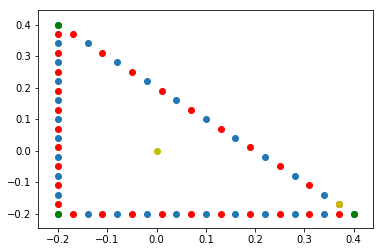To do this we introduce the Helmholtz fundamental solution: $$\Phi_k(\vec{x},\vec{y}) = \frac{i}{4} H_0^1(|\vec{x}-\vec{y}|),\qquad\qquad \forall \vec{x},\vec{y} \in \mathbb{R}^2 \; such\; that\;\vec{x}\ne \vec{y} .$$ Any linear combination of the form $\sum_{j} \psi_j \Phi_k(\cdot,\vec{y_j})$, where $\vec{y_j}\in\overline{\Omega_{-}}$, is a radiating Helmholtz solution in $\Omega_{+}$. We can take a continuous linear combination, focused on $\vec{y_j}\in \partial \Omega_{-}$, to obtain a radiating Helmholtz solution: $$\label{eq:BIO}\mathcal{S}\psi (\vec{x}) = \int_{\partial\Omega_{-}} \psi(\vec{y})\Phi_k(\vec{x},\vec{y})ds(\vec{y})\qquad\qquad \vec{x}\in \Omega_{+}$$ the operator $\mathcal{S}$ is called Helmholtz single layer potential. We similarly define the single layer operator as follow: $$\label{eq:BIE}S\psi (\vec{x}) = \int_{\partial\Omega_{-}} \psi(\vec{y})\Phi_k(\vec{x},\vec{y})ds(\vec{y})\qquad\qquad \vec{x}\in \partial\Omega_{-}$$ to find the potential $\psi$ that satisfy the previous equation we use a collocation method. In a collocation method we fix a discrete space $V_h\subset H^{-\frac{1}{2}}(\partial\Omega_{-})$, in particular since $L^2(\partial\Omega_{-})$ is contained in $H^{-\frac{1}{2}}(\partial\Omega_{-})$ we like for $V_h$ to accommodate discontinuous functions. To build $V_h$ we divide $\partial\Omega_{-}$ in a number of elements $K_j$, then we define $V_h$ as: $$V_h = <\varphi_1,\cdots, \varphi_{NDoF}>,\qquad\qquad \varphi_j(s) = \begin{cases} 1\Leftrightarrow s \in K_j\\ 0\Leftrightarrow s \in \bigcup_{i\ne j}K_i \end{cases}$$ we can rewrite the second integral equation assuming that $\psi$ lives in $V_h$, i.e. $\psi = \sum_{j}\vec{\psi}_j\varphi_j$ : $$\sum_{j} \vec{\psi}_j \int_{\partial\Omega_{-}}\Phi_k(\vec{x},\vec{y})\varphi_j(\vec{y}) ds(\vec{y})=g(\vec{x})$$ we impose the previous equation on the midpoints of the segments $K_j$ to obtain the following linear system: $$A\vec{x} = \vec{b}\\ A_{ij} = \int_{\partial\Omega_{-}}\Phi_k(\vec{m_i},\vec{y})\varphi_j(\vec{y}) ds(\vec{y})=\int_{K_j}\Phi_k(\vec{m_i},\vec{y})ds(\vec{y})\\ b_i = g(\vec{m}_i)$$ where $\vec{m}_i$ is the midpoint of the segment $K_i$. Solving the linear system we obtain the vector $\vec{\psi}$, and we can use first integral equation to compute the scattered wave:

$$u_{st}(\vec{x}) = S\psi (\vec{x}) = \int_{\partial\Omega_{-}} \psi(\vec{y})\Phi_k(\vec{x},\vec{y})ds(\vec{y})=\int_{\partial\Omega_{-}}\sum_j\psi_j\varphi_j(\vec{y})\Phi_k(\vec{x},\vec{y})ds(\vec{y})=\\\sum_{j}\psi_j\int_{K_j}\Phi_k(\vec{x},\vec{y})ds(\vec{y})$$

In :
#We here define a planar wave in the direction d=(0.5sqrt(3),0.5) with k=10.
# u(x) = exp(i(x°d)) = exp(ik*rho*|d|*cos(theta-pi/6))
k=30
def PW(Angle):
pw = exp(1j*k*sqrt(x**2+y**2)*sqrt((0.5*sqrt(3))**2+0.25)*cos(theta-Angle));
return pw;

N = 3*M; #Full number of segments
A = np.zeros((N,N),complex) #Init of the stifness matrix
F = np.zeros((N,1),complex) #Init of vector b, Ax=b.

for j in range(0,N):
for i in range(0,N):
#Computing Single Layer Potential with as one variable the mid points.
HelmholtzSLP = CoefficientFunction(0.25*1j*sp.hankel1(z=k*sqrt((TPnts2[j]-x)**2+(TPnts2[j]-y)**2),v=0))
#Integrating SLP to create the stifness matrix.
A[i,j]= Integrate(HelmholtzSLP,mesh,definedon=mesh.Boundaries("m"+str(2*(i+1)-1)),order=20)+Integrate(HelmholtzSLP,mesh,definedon=mesh.Boundaries("m"+str(2*(i+1))),order=20);
if i in range(M,2*M):
A[i,j] = (1/sqrt(2))*A[i,j]; #Correction factor due to NGSOLVE integration.
mip = mesh(TPnts2[j],TPnts2[j]); #Defining mid point as mesh element
#j-th components of b is the value of the planaer wave in the mid point
F[j] = PW(pi/3)(mip);
print("Matrix Conditioning: ", np.linalg.cond(A))
Psi = np.linalg.solve(A,F) #Computing x

gamma=0.6 #Scaling factor of the (0,0)-(0,1)-(1,0) triangle.

def SLO(Psi):
#We integrate using a mid point quadrature rule to obtain a function in one variable.
u = 0*x+0*y; #Init u
N=len(Psi);
M =int(len(Psi)/3);
for j in range(0,N):
#We divide the integration among the three edge of the triangle
if j in range(0,M):
h = (gamma)*(1/M);
plt.scatter(TPnts2[j],TPnts2[j],c="y")
HelmholtzSLP = CoefficientFunction(0.25*1j*sp.hankel1(z=k*sqrt((TPnts2[j]-x)**2+(TPnts2[j]-y)**2),v=0))
elif j in range(2*M,N):
plt.scatter(TPnts2[j],TPnts2[j],c="g")
h = (gamma)*(1/M);
HelmholtzSLP = CoefficientFunction(0.25*1j*sp.hankel1(z=k*sqrt((TPnts2[j]-x)**2+(TPnts2[j]-y)**2),v=0))
elif j in range(M,2*M):
plt.scatter(TPnts2[j],TPnts2[j],c="r")
dt = 1#sqrt(2) #On the hypotenuse we have a factor sqrt(2) to correct.
h = (gamma/M)*dt
HelmholtzSLP = CoefficientFunction(0.25*1j*sp.hankel1(z=k*sqrt((TPnts2[j]-x)**2+(TPnts2[j]-y)**2),v=0))
u -=h*Psi[j]*HelmholtzSLP;
return u;

Ust = SLO(Psi)
Uin = PW(pi/3)
Utot = Ust+Uin;
Draw(Uin,mesh,'Uin')

Draw(Ust,mesh,'Ust')
Draw(Utot,mesh,'Utot')

triag = SplineGeometry()
Del = (gamma)*(2/M);
triagpnts =[(-0.2+Del,-0.2+Del),(-0.2+Del,0.4-Del),(0.4-Del,-0.2+Del)]
p1,p2,p3= [triag.AppendPoint(*pnt) for pnt in triagpnts]
triag.Append(['line', p1, p3], bc='bottom', leftdomain=1, rightdomain=0)
triag.Append(['line', p3, p2], bc='right', leftdomain=1, rightdomain=0)
triag.Append(['line', p2,p1], bc='left', leftdomain=1, rightdomain=0)

MIP = TriagMesh(-0.2+Del,-0.2+Del);
Ust = SLO(Psi)
Uin = PW(pi/3)
u = Ust+Uin;
Draw(u,TriagMesh,'u')
print(u(MIP))
Matrix Conditioning:  24.76304609462367
(-0.02079322710624032+0.0010457494082059815j)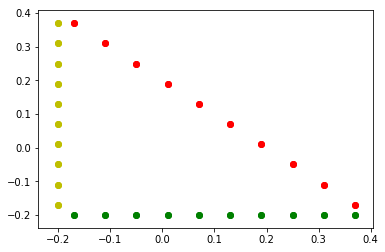We now study the evolution of the quantity $|u_{St}+u_{In}|$ in the inside of the scatter knowing that inside of the scatterer $u_{St}+u_{In}$ is null.

In :
err = [] #Init. vector of abs(Ust+Uin)
errI = [] #Init. vector of Integral Ust+Uin
H = [] #Init. of the diameter vector
IT = list(range(4,50,2));
#Computing Ust and Uin for each M.
for M in IT:
geo = SplineGeometry()
gamma = 0.6;
def l(x):
return -x+1;

pnts=[];
for i in range(0,M):
pnts.append((0,i/M));
for i in range(0,M):
pnts.append((i/M,l(i/M)))
for i in range(0,M):
pnts.append(((M-i)/M,0));
TPnts = [(gamma*(p-(1/3)),gamma*(p-(1/3)))for p in pnts]
P = [geo.AppendPoint(*pnt) for pnt in TPnts]

Curves = [[["line",P[i],P[i+1]],"l"+str(int(i))] for i in range(0,len(pnts)-1)]
Curves.append([["line",P[len(pnts)-1],P],"l"+str(int(len(pnts)-1))])

pnts2=[];
for j in range(0,3*M):
if j in range(0,M):
pnts2.append((pnts[j],pnts[j]+(0.5/M)));
elif j in range(2*M,3*M):
pnts2.append((pnts[j]-(0.5/M),pnts[j]));
else:
pnts2.append((pnts[j]+(0.5/M),pnts[j]-(0.5/M)))

TPnts2 = [(gamma*(p-(1/3)),gamma*(p-(1/3)))for p in pnts2]

P2 = [geo.AppendPoint(*pnt) for pnt in TPnts2]

Curves = []
for i in range(0,len(pnts)-1):
Curves.append([["line",P[i],P2[i]],"m"+str(2*int(i+1)-1)])
Curves.append([["line",P2[i],P[i+1]],"m"+str(2*int(i+1))])
Curves.append([["line",P[len(pnts)-1],P2[len(pnts)-1]],"m"+str(2*(len(pnts2))-1)])
Curves.append([["line",P2[len(pnts)-1],P],"m"+str(2*(len(pnts2)))])

[geo.Append(c,bc=bc) for c,bc in Curves]

mesh = Mesh(geo.GenerateMesh(maxh=0.5/M))

rho = sqrt(x**2+y**2);
theta = atan2(y,x)

N = 3*M;
A = np.zeros((N,N),complex)
F = np.zeros((N,1),complex)

for j in range(0,N):
for i in range(0,N):
HelmholtzSLP = CoefficientFunction(0.25*1j*sp.hankel1(z=k*sqrt((TPnts2[j]-x)**2+(TPnts2[j]-y)**2),v=0))
A[i,j]= Integrate(HelmholtzSLP,mesh,definedon=mesh.Boundaries("m"+str(2*(i+1)-1)),order=10)+Integrate(HelmholtzSLP,mesh,definedon=mesh.Boundaries("m"+str(2*(i+1))),order=10);
if i in range(M,2*M):
A[i,j] = (1/sqrt(2))*A[i,j];#Correction factor due to NGSOLVE integration.
mip = mesh(TPnts2[j],TPnts2[j]);
F[j] = PW(pi/3)(mip);
print("Matrix Conditioning: ", np.linalg.cond(A))
Psi = np.linalg.solve(A,F);

triag = SplineGeometry()
Del = (gamma)*(2/M);
triagpnts =[(-0.2,-0.2),(-0.2,0.4),(0.4,-0.2)]
p1,p2,p3= [triag.AppendPoint(*pnt) for pnt in triagpnts]
triag.Append(['line', p1, p3], bc='bottom', leftdomain=1, rightdomain=0)
triag.Append(['line', p3, p2], bc='right', leftdomain=1, rightdomain=0)
triag.Append(['line', p2,p1], bc='left', leftdomain=1, rightdomain=0)
TriagMesh = Mesh(triag.GenerateMesh(maxh=0.02))

MIP = TriagMesh(-0.2+Del,-0.2+Del);
Ust = SLO(Psi)
Uin = PW(pi/3)
u = Ust+Uin;
print("IT: ",M," Raggio: ",0.5/M," Sum of Ust and Uin in (0,0): ",abs(u(MIP))," Integral: ",abs(Integrate(u,TriagMesh,order=10)))
err.append(abs(u(MIP)))
errI.append(abs(Integrate(u,TriagMesh,order=10)))
H.append(0.5/M);

Matrix Conditioning:  5.6106334818508365
IT:  4  Raggio:  0.125  Sum of Ust and Uin in (0,0):  1.007722586432051  Integral:  0.004172826209686019
Matrix Conditioning:  56.24443970847265
IT:  6  Raggio:  0.08333333333333333  Sum of Ust and Uin in (0,0):  0.1533159268185393  Integral:  0.0029870160011187413
Matrix Conditioning:  31.325733288844077
IT:  8  Raggio:  0.0625  Sum of Ust and Uin in (0,0):  0.09576164905382228  Integral:  0.001496199440647954
Matrix Conditioning:  23.6149032286072
IT:  10  Raggio:  0.05  Sum of Ust and Uin in (0,0):  0.022825852450449973  Integral:  0.0008349134463713026
Matrix Conditioning:  21.23472579503481
IT:  12  Raggio:  0.041666666666666664  Sum of Ust and Uin in (0,0):  0.006100292162710276  Integral:  0.0005711258767915209
Matrix Conditioning:  20.473598218291915
IT:  14  Raggio:  0.03571428571428571  Sum of Ust and Uin in (0,0):  0.005997282727116005  Integral:  0.0004205851833839539
Matrix Conditioning:  20.169739449289015
IT:  16  Raggio:  0.03125  Sum of Ust and Uin in (0,0):  0.00770408603735146  Integral:  0.00032308079422956645
Matrix Conditioning:  20.03957051387714
IT:  18  Raggio:  0.027777777777777776  Sum of Ust and Uin in (0,0):  0.008850008202893755  Integral:  0.00025637056878895
Matrix Conditioning:  19.985655153480916
IT:  20  Raggio:  0.025  Sum of Ust and Uin in (0,0):  0.009374330217802299  Integral:  0.00020687279192416444
Matrix Conditioning:  19.96804772858423
IT:  22  Raggio:  0.022727272727272728  Sum of Ust and Uin in (0,0):  0.009446089082701113  Integral:  0.0001741669566814307
Matrix Conditioning:  19.96847691257227
IT:  24  Raggio:  0.020833333333333332  Sum of Ust and Uin in (0,0):  0.009243031820518229  Integral:  0.0001468375947051803
Matrix Conditioning:  19.977951419978076
IT:  26  Raggio:  0.019230769230769232  Sum of Ust and Uin in (0,0):  0.008894261368819989  Integral:  0.0001243769306874412
Matrix Conditioning:  19.99182430393813
IT:  28  Raggio:  0.017857142857142856  Sum of Ust and Uin in (0,0):  0.008481151487831275  Integral:  0.0001078839000408911
Matrix Conditioning:  20.007637966920335
IT:  30  Raggio:  0.016666666666666666  Sum of Ust and Uin in (0,0):  0.00805113839151714  Integral:  9.188870362971513e-05
Matrix Conditioning:  20.08268140410223
IT:  32  Raggio:  0.015625  Sum of Ust and Uin in (0,0):  0.007630168293875003  Integral:  8.365506721556845e-05
Matrix Conditioning:  21.424495066489747
IT:  34  Raggio:  0.014705882352941176  Sum of Ust and Uin in (0,0):  0.0072313318663903186  Integral:  7.418171716914986e-05
Matrix Conditioning:  22.7646059643929
IT:  36  Raggio:  0.013888888888888888  Sum of Ust and Uin in (0,0):  0.0068603069022546955  Integral:  6.675484331062248e-05
Matrix Conditioning:  24.101409843736576
IT:  38  Raggio:  0.013157894736842105  Sum of Ust and Uin in (0,0):  0.0065186388088349775  Integral:  6.11217858921818e-05
Matrix Conditioning:  25.435497664954724
IT:  40  Raggio:  0.0125  Sum of Ust and Uin in (0,0):  0.006205668557352301  Integral:  5.424954240558661e-05
Matrix Conditioning:  26.7689149617162
IT:  42  Raggio:  0.011904761904761904  Sum of Ust and Uin in (0,0):  0.005919644896235746  Integral:  4.934826164503913e-05
Matrix Conditioning:  28.100007426434797
IT:  44  Raggio:  0.011363636363636364  Sum of Ust and Uin in (0,0):  0.005658353759041295  Integral:  4.4190658225278294e-05
Matrix Conditioning:  29.42905140066135
IT:  46  Raggio:  0.010869565217391304  Sum of Ust and Uin in (0,0):  0.005419465177826859  Integral:  4.0802095944816064e-05
Matrix Conditioning:  30.75628142835058
IT:  48  Raggio:  0.010416666666666666  Sum of Ust and Uin in (0,0):  0.005200716470461735  Integral:  3.7830383616309985e-05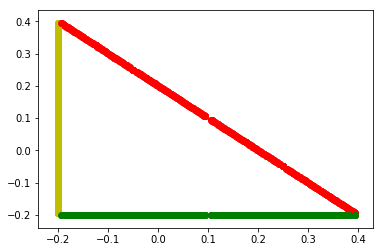In :
#Plot the graph of err vs H
plt.figure()
plt.plot(H,err,"*-")
plt.plot(H,H,"r--")

plt.title("Absolute Value Sum Of Scattered And Incoming Wave")
plt.ylabel("|Ust + Uin| in a point of the scatterer")
plt.xlabel("h")

plt.yscale("log")
plt.xscale("log")

print("Factor of convergence: ",log(err[-2]/err[-10])/log(H[-2]/H[-10]))

#Plot the graph of err vs H
plt.figure()
plt.plot(H,errI,"*-")
plt.plot(H,H,"r--")

plt.title("Absolute Value Sum Of Scattered And Incoming Wave")
plt.ylabel("Integral of Ust + Uin")
plt.xlabel("h")

plt.yscale("log")
plt.xscale("log")

print("Factor of convergence: ",log(errI[-1]/errI[-10])/log(H[-1]/H[-10]))
Factor of convergence:  0.9260074954675956
Factor of convergence:  1.8882099354486628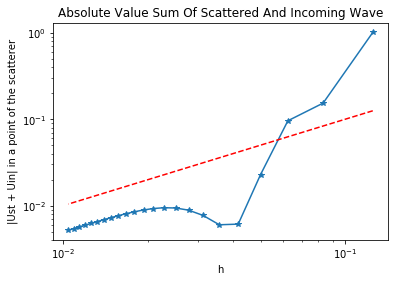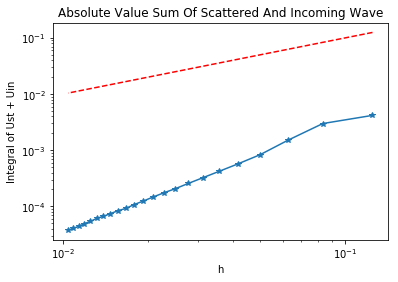In :
from IPython.display import Video

Video("SSSP2.mp4")
Out:

FEM and Truncated Problem¶

We now take a look at a different method aimed at solving the SSSP using finite element methods (FEM) rather then boundary element methods (BEM). The exterior Dirichlet problem is equivalent to the following PDE on a truncated domain $\Omega = B_R(\vec{0})\cap \Omega_{+}$: $$\Delta u + k^2 u = 0\; in\; \Omega\\ \gamma^{+} u = g\; on\; \partial\Omega_{-}\\ DtN(\gamma^{+}u) -\partial_n u = 0 \; on \; \partial B_R(\vec{0})$$ where the DtN is the operator defined as follow.

Definition¶

Given a function $g:\partial B_R(\vec{0})\to \mathbb{R}$ we can decompose $g$ in circular harmonics series, i.e Fourier series: $$g(\theta) = \sum_{l\in \mathbb{Z}} v_l e^{il\theta}$$ the DtN operator acts as follow: $$DtN(g) = \sum_{l\in\mathbb{Z}} \frac{k H_l^{(1)}(kR)}{H_l^{(1)}(kR)} v_l e^{il\theta}$$ this can be written in variational form as follow: $$Find\; u\in H^{1}_{0}(\Omega) \; such \; that\; A(u,v) = F(v)\; \forall v \in H^{1}_{0}(\Omega)\\ A(u,v) = \int_\Omega (\nabla u \overline{\nabla v} -k^2u\overline{v})\,d\vec{x} - \int_{\partial B_R} DtN(\gamma^{+}u)(\gamma^{+}v)\,ds\\ F(v) = \int_\Omega f\overline{v}\,d\vec{x}$$ as $R$ grows to infinity we can replace the previous problem with the following: $$Find\; u\in H^{1}_{0}(\Omega) \; such \; that\; A(u,v) = F(v)\; \forall v \in H^{1}_{0}(\Omega)\\ A(u,v) = \int_\Omega (\nabla u \overline{\nabla v} -k^2u\overline{v})\,d\vec{x} - ik^2\int_{\partial B_R} u\overline{v},ds\\ F(v) = \int_\Omega f\overline{v}\,d\vec{x}$$ Here we have imposed some conditions that mimics the radiating condition with an impedance condition that is equivalent to the radiating condition when $R$ grows to infinity.

In [ ]:
geo = SplineGeometry()
########################
#  TRIANGLE SCATTERER
########################
gamma = 0.6;
def l(x):
return -x+1;

M = 10;
N = 3*M

pnts=[];
for i in range(0,M+1):
pnts.append((0,i/M));
for i in range(1,M):
pnts.append((i/M,l(i/M)))
for i in range(0,M):
pnts.append(((M-i)/M,0));
TPnts = [(gamma*(p-(1/3)),gamma*(p-(1/3)))for p in pnts]
P = [geo.AppendPoint(*pnt) for pnt in TPnts]

Curves = [[["line",P[i],P[i+1]],"l"+str(int(i))] for i in range(0,len(pnts)-1)]
Curves.append([["line",P[len(pnts)-1],P],"l"+str(int(len(pnts)-1))])

pnts2=[];
for j in range(0,3*M):
if j in range(0,M):
pnts2.append((pnts[j],pnts[j]+(0.5/M)));
elif j in range(2*M,3*M):
pnts2.append((pnts[j]-(0.5/M),pnts[j]));
else:
pnts2.append((pnts[j]+(0.5/M),pnts[j]-(0.5/M)))

TPnts2 = [(gamma*(p-(1/3)),gamma*(p-(1/3)))for p in pnts2]

P2 = [geo.AppendPoint(*pnt) for pnt in TPnts2]

Curves = []
for i in range(0,len(pnts)-1):
Curves.append([["line",P[i],P2[i]],"m"+str(2*int(i+1)-1)])
Curves.append([["line",P2[i],P[i+1]],"m"+str(2*int(i+1))])
Curves.append([["line",P[len(pnts)-1],P2[len(pnts)-1]],"m"+str(2*(len(pnts2))-1)])
Curves.append([["line",P2[len(pnts)-1],P],"m"+str(2*(len(pnts2)))])

[geo.Append(c,bc=bc) for c,bc in Curves]

mesh = Mesh(geo.GenerateMesh(maxh=0.5/M)) #Meshing ...
#Defining polar coordinates.
rho = sqrt(x**2+y**2);
theta = atan2(y,x)
In [ ]:
#We here define a planar wave in the direction d=(0.5sqrt(3),0.5) with k=10.
# u(x) = exp(i(x°d)) = exp(ik*rho*|d|*cos(theta-pi/6))
k=10
def PW(Angle):
pw = exp(1j*k*sqrt(x**2+y**2)*sqrt((0.5*sqrt(3))**2+0.25)*cos(theta-Angle));
return pw;
Uin = PW(pi/3)
Draw(Uin,mesh,'Uin'); #We plot Uin
#We define a H1 complex space with Dirichlet boundary condition on the circle edge.
fes = H1(mesh, order=1, complex=True,dirichlet="|".join(["m"+str(i) for i in range(1,2*N+1)]))
u, v = fes.TnT() #Trial and test functions

#We define the bilinear form.
a = BilinearForm(fes)
a += -k*1j*u*v * ds("outer") #Impedence condition
a.Assemble()

#Linear application
f = LinearForm(fes)
f += 0 * v * dx
f.Assemble()

uh = GridFunction(fes, name="uh") #Init. Ust
uBND = GridFunction(fes, name="UinBnd") #Init. Uin on the boundary
uh.Set(Uin,BND) #Setting Boundary condition
uBND.Set(Uin,BND) #Setting Boundary condition
Draw(uBND,mesh,"UinBND") #Plotting Uin on the boundary

#Solving fot Ust
r = f.vec.CreateVector()
r.data = f.vec - a.mat * uh.vec
uh.vec.data += a.mat.Inverse(freedofs=fes.FreeDofs()) * r
#Drawing Ust and Ust-Uin
Draw(uh,mesh,'uh')
Draw(uh-Uin,mesh,'utot')
In :
from IPython.display import Video

Video("SSSP3.mp4")
Out: